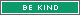1086 Posts
  Prev «  14 , 15 , 16 , 17 , 18  Next »   |

## Subject: Win 10 Geek Gold for Rolling the Geek Roller! (Roll 1947 to win)d2000 = (1753) = 1753
• [+] Dice rolls
• [+]
• 1477979. CrazyCatman
• d2000 =
• (1753) =
• 1753
• Sun Jun 23, 2019 8:23 am
1d2000 = (1569) = 1569
• [+] Dice rolls
• [+]
• 1477995. Jrtolf
• 1d2000 =
• (1569) =
• 1569
• Sun Jun 23, 2019 12:31 pm
Rose
United States
New York City
NY
"I do what I do because it’s right! Because it’s decent! And above all, it’s kind! It’s just that… Just kind." -Dr. Who"And forget not that the earth delights to feel your bare feet and the winds long to play with your hair." - Khalil Gibran
In military time, I will finally feel awake at 1d2000 = (1313) = 1313
• [+] Dice rolls
• [+]
• 1478009. anjulka
• 1d2000 =
• (1313) =
• 1313
• In military time, I will finally feel awake at
• Sun Jun 23, 2019 3:16 pm
d2000 = (240) = 240
• [+] Dice rolls
• [+]
• 1478030. bulldog93
• d2000 =
• (240) =
• 240
• Sun Jun 23, 2019 4:47 pm
You drink from an unknown pool of water. You feel invigorated! Gain d2000 = (1723) = 1723 experience.
• [+] Dice rolls
• [+]
• 1478081. wizcreations
• d2000 =
• (1723) =
• 1723
• Sun Jun 23, 2019 10:57 pm
1d2000 = (1395) = 1395
• [+] Dice rolls
• [+]
• 1478108. ghostpants
• 1d2000 =
• (1395) =
• 1395
• Mon Jun 24, 2019 1:26 am
I am d2000 = (1245) = 1245% sure this will work!
• [+] Dice rolls
• [+]
• 1478128. Jlerpy
• d2000 =
• (1245) =
• 1245
• Mon Jun 24, 2019 3:56 am
d2000 = (1212) = 1212
• [+] Dice rolls
• [+]
• 1478138. CrazyCatman
• d2000 =
• (1212) =
• 1212
• Mon Jun 24, 2019 7:04 am
1d2000 = (1697) = 1697
• [+] Dice rolls
• [+]
• 1478165. Jrtolf
• 1d2000 =
• (1697) =
• 1697
• Mon Jun 24, 2019 9:25 am
d2000 = (507) = 507
• [+] Dice rolls
• [+]
• 1478413. bulldog93
• d2000 =
• (507) =
• 507
• Mon Jun 24, 2019 9:51 pm
1d2000 = (1979) = 1979
• [+] Dice rolls
• [+]
• 1478424. ghostpants
• 1d2000 =
• (1979) =
• 1979
• Mon Jun 24, 2019 10:37 pm
Rose
United States
New York City
NY
"I do what I do because it’s right! Because it’s decent! And above all, it’s kind! It’s just that… Just kind." -Dr. Who"And forget not that the earth delights to feel your bare feet and the winds long to play with your hair." - Khalil Gibran
No, I will not buy you 1d2000 = (174) = 174 blue marbles.
• [+] Dice rolls
• [+]
• 1478459. anjulka
• 1d2000 =
• (174) =
• 174
• No, I will not buy you
• Tue Jun 25, 2019 1:01 am
How about d2000 = (1386) = 1386 red marbles?
• [+] Dice rolls
• [+]
• 1478502. wizcreations
• d2000 =
• (1386) =
• 1386
• Tue Jun 25, 2019 6:02 am
wizcreations wrote:
How about wizcreations previously rolled d2000 = (1386) = 1386 ( ) red marbles?
Don't be greedy!
Then if you are nice she will give you d2000 = (1636) = 1636 green marbles
• [+] Dice rolls
• [+]
• 1478531. CrazyCatman
• d2000 =
• (1636) =
• 1636
• Then if you are nice she will give you
• Tue Jun 25, 2019 10:27 am
I think I'll roll a 1d2000 = (1462) = 1462 today.
• [+] Dice rolls
• [+]
• 1478538. Jrtolf
• 1d2000 =
• (1462) =
• 1462
• I think I'll roll a
• Tue Jun 25, 2019 12:09 pm
Jrtolf wrote:
Jrtolf previously rolled 1d2000 = (1462) = 1462 (I think I'll roll a ) today.
Nice! I also plan on rolling a 1d2000 = (1646) = 1646 today
• [+] Dice rolls
• [+]
• 1478634. ColdFrog
• 1d2000 =
• (1646) =
• 1646
• Tue Jun 25, 2019 3:42 pm
d2000 = (67) = 67
• [+] Dice rolls
• [+]
• 1478735. GeoffreyB
• d2000 =
• (67) =
• 67
• Tue Jun 25, 2019 7:48 pm
d2000 = (1525) = 1525
• [+] Dice rolls
• [+]
• 1478750. bulldog93
• d2000 =
• (1525) =
• 1525
• Tue Jun 25, 2019 8:30 pm
1d2000 = (490) = 490
• [+] Dice rolls
• [+]
• 1478886. ghostpants
• 1d2000 =
• (490) =
• 490
• Wed Jun 26, 2019 1:08 am
I plan to save \$ d2000 = (1183) = 1183 in the Steam sale.
• [+] Dice rolls
• [+]
• 1478889. wizcreations
• d2000 =
• (1183) =
• 1183
• Wed Jun 26, 2019 1:40 am
d2000 = (4) = 4
• [+] Dice rolls
• [+]
• 1479015. CrazyCatman
• d2000 =
• (4) =
• 4
• Wed Jun 26, 2019 11:29 am
1d2000 = (1116) = 1116
• [+] Dice rolls
• [+]
• 1479042. Jrtolf
• 1d2000 =
• (1116) =
• 1116
• Wed Jun 26, 2019 12:28 pm
Here we go!D2000 = (1005) = 1005
• [+] Dice rolls
• [+]
• 1479056. Jlerpy
• D2000 =
• (1005) =
• 1005
• Wed Jun 26, 2019 12:54 pm
I'm feelin' lucky! 1d2000 = (856) = 856
• [+] Dice rolls
• [+]
• 1479099. MABBY
• 1d2000 =
• (856) =
• 856
• I'm feelin' lucky!
• Wed Jun 26, 2019 2:37 pm
d2000 = (731) = 731
• [+] Dice rolls
• [+]
• 1479146. bulldog93
• d2000 =
• (731) =
• 731
• Wed Jun 26, 2019 4:48 pm
  Prev «  14 , 15 , 16 , 17 , 18  Next »   |